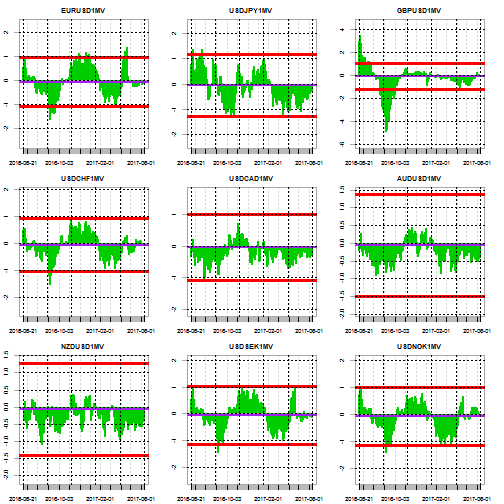# G10 FX Implied Volatilities: Cheap or Expensive ?

The following report provides a granular analysis of implied volatilities within G10 FX. I use primarily the same formatting than for my G10FX positioning report to estimate how extended the 1-month FX implied volatilities are over various time horizon.

The first set of charts shows the historical T-stat of the 1-day changes in 1-month implied volatilities over a rolling period of 61-days. This is my statistical metric to quantify how stretched the implied volatilities are, but clearly other time period could be used as shown further down on in that report. The purple line represents the median value since 1996 and the red lines represent the 95% confidence intervals. Therefore if the value is above or below those the deviation of the given implied volatility should be deemed as atypical relative to what would be expected under a normal distribution (I am not saying that implied volatilities have a normal behaviour to be clear….) and therefore overbought/oversold.The below charts shows the current implied volatilities relative to their historical distributions since 1996. Once again the red lines delimit the 95% confidence intervals and the purple line the median value. The blue line indicates the most current level of 1-month implied volatility.Finally the below shows a stretch map of the T-Stats to help visualise how much implied volatilities have departed from their equilibrium levels over time horizons ranging from 1-month to 6-month. The bigger the square the most significant the observed upside (Green) or downside (Red) of the implied volatility over the given period.